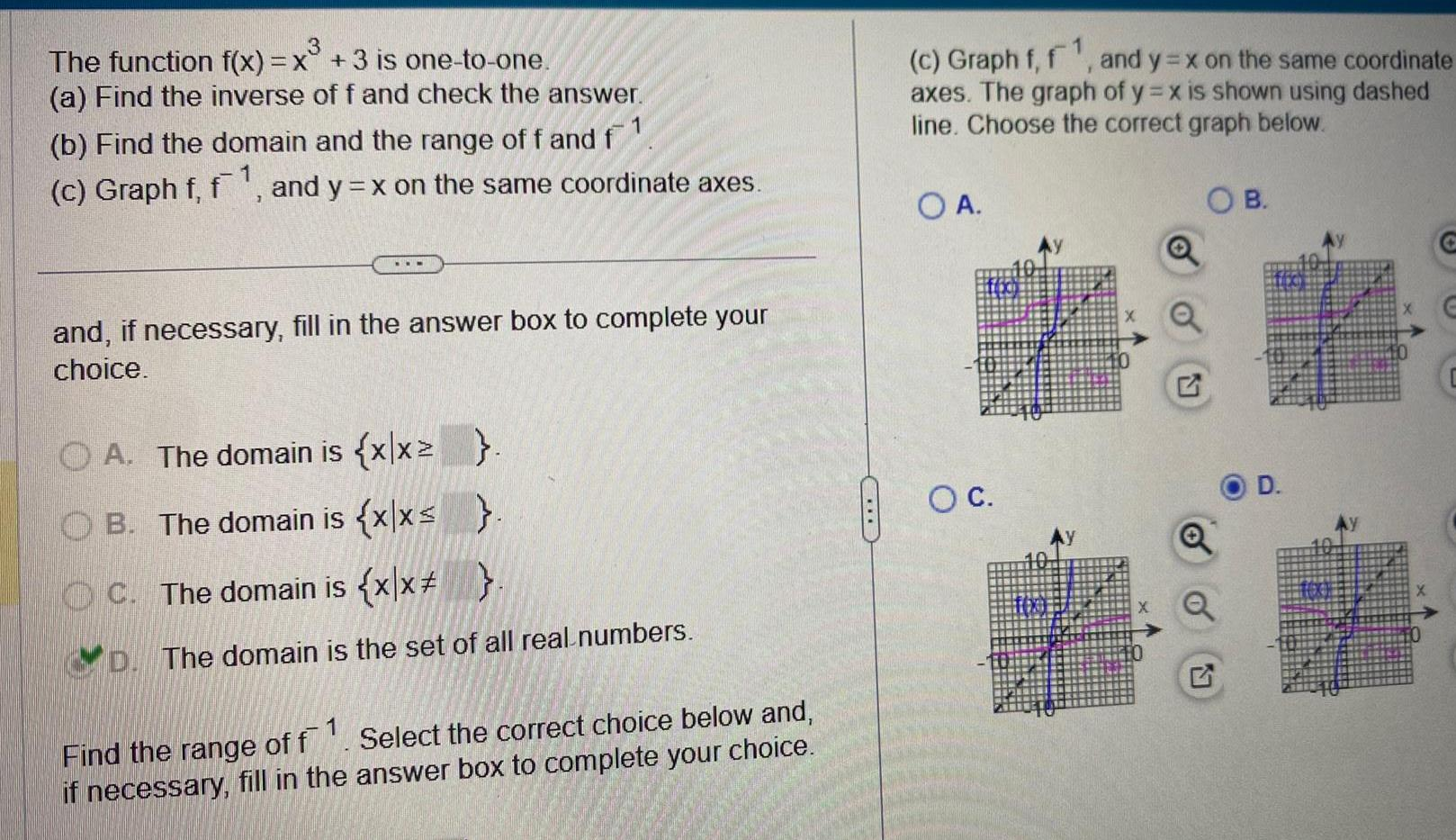Question:

# 3 The function f x x 3 is one to one a Find the inverse of f

Last updated: 11/20/20233 The function f x x 3 is one to one a Find the inverse of f and check the answer b Find the domain and the range of f and f c Graph f f1 and y x on the same coordinate axes and if necessary fill in the answer box to complete your choice A The domain is x x B The domain is x x C The domain is x x D The domain is the set of all real numbers Find the range of f if necessary fill in the Select the correct choice below and answer box to complete your choice 1 c Graph f f and y x on the same coordinate axes The graph of y x is shown using dashed line Choose the correct graph below OA O C B D E